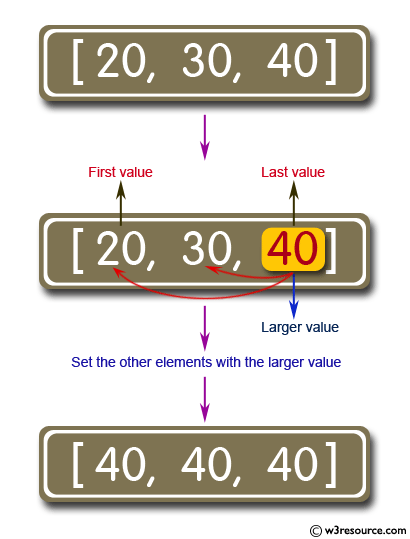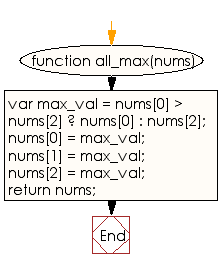# JavaScript: Find the larger value between the first or last and set all the other elements with that value

## JavaScript Basic: Exercise-74 with Solution

Write a JavaScript program to find the larger value between the first or last and set all the other elements with that value. Display the new array.

Pictorial Presentation:Sample Solution:

HTML Code:

``````<!DOCTYPE html>
<html>
<meta charset="utf-8">
<meta name="viewport" content="width=device-width">
<title>JavaScript program to find the larger value between the first or last and set all the other elements with that value.  Display the new array.</title>
<body>

</body>
</html>
```
```

JavaScript Code:

``````function all_max(nums)
{
var max_val = nums > nums ? nums : nums;

nums = max_val;
nums = max_val;
nums = max_val;

return nums;
}
console.log(all_max([20, 30, 40]));
console.log(all_max([-7, -9, 0]));
console.log(all_max([12, 10, 3]));
```
```

Sample Output:

```[40,40,40]
[0,0,0]
[12,12,12]
```

Flowchart:ES6 Version:

``````function all_max(nums)
{
const max_val = nums > nums ? nums : nums;

nums = max_val;
nums = max_val;
nums = max_val;

return nums;
}
console.log(all_max([20, 30, 40]));
console.log(all_max([-7, -9, 0]));
console.log(all_max([12, 10, 3]));
``````

Live Demo:

See the Pen JavaScript - Find the larger value between the first or last - basic-ex-74 by w3resource (@w3resource) on CodePen.

What is the difficulty level of this exercise?

Test your Programming skills with w3resource's quiz.

﻿

## JavaScript: Tips of the Day

Checks if a string is an anagram of another string (case-insensitive, ignores spaces, punctuation and special characters)

Example:

```const isAnagram = (str1, str2) => {
const normalize = str =>
str
.toLowerCase()
.replace(/[^a-z0-9]/gi, '')
.split('')
.sort()
.join('');
return normalize(str1) === normalize(str2);
};
console.log(isAnagram('iceman', 'cinema')); // true
```

Output:

```true
```